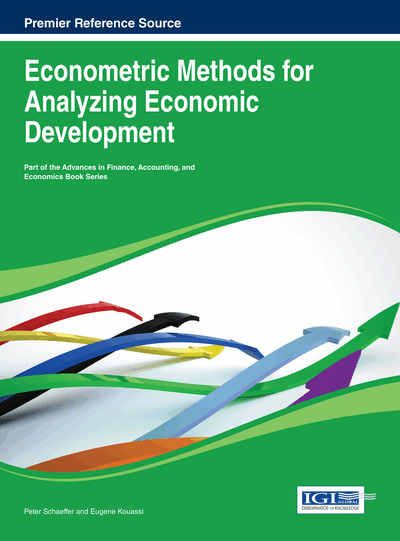# Simulating Impacts on Regional Economies: A Modeling Alternative

Guy R. West (University of Sunshine Coast, Australia) and Randall W. Jackson (West Virginia University, USA)
DOI: 10.4018/978-1-4666-4329-1.ch009

## Abstract

Practitioners and academics apply a range of regional economic models for impacts assessment. These models extend from a simple economic base through to input-output and econometric models and computable general equilibrium models. All such models have strengths and weaknesses. Dimensions of which impact assessment models are often compared include level of industry detail, data availability, and complexity of behaviour modelled. This chapter presents a model for Simulating Impacts on Regional Economies (SIRE) that occupies an intermediate position between Input-Output (IO), arguably the most widely used model for regional impacts assessments, and Computable General Equilibrium (CGE) models. With greater behavioural detail than the typical regional IO model, the SIRE model incorporates many of the features of CGE models without enforcing the strictly linear behavioural relationships of IO. Like most CGE models, the simulation framework presented here borrows a subset of parameters from an existing econometric model for the same region. The SIRE model falls short, however, of the complexity of capturing the full range of behaviours of CGE models.
Chapter Preview
Top

## 1. Introduction

Economic modeling at the regional and small area level is restricted by model and data availability. Often, resource and time limitations preclude the construction of complex models such as Computable General Equilibrium (CGE) models, and in fact there are arguments to suggest that building a CGE model for a small region, while not invalid, may not be a very efficient use of resources in the context of the tradeoff between increased complexity and increased data ”fuzziness” (West, 2002).

Input-Output (IO), the old workhorse of the regional modeler, is usually used in such cases since it really provides the only practical option to planners. While many have forecast the end of the simple input-output model due to its inadequacies, it has proved surprisingly resilient.

The assumptions of the input-output model are concerned almost entirely with the nature of production. Inter-industry models are based on the premise that it is possible to divide all productive activities in an economy into sectors or industries whose inter-relations can be meaningfully expressed as a set of equations. The crucial assumption in the IO model1 is that the money value of goods and services delivered by an industry to other producing sectors is a linear and homogeneous function of the output level of the purchasing industry with supply being infinitely elastic.

This linearity assumption clearly lays simple IO models open to valid criticism. It implies a strict proportional relationship between input coefficients and output; for example, income coefficients are average propensities and employment coefficients reflect average labor productivity rates. In impact studies, this property can lead to an overestimation of the flow-on (multiplier) effects, particularly if the initial impacts are relatively modest. For example, many industries can increase output in the short term without corresponding proportional increases in wage costs and employment, particularly if there is slack capacity.

There have been various attempts to model non-linearity in the input demand structure. Evans (1954) was one of the first to generalize the input-output model by allowing the input coefficients to vary with the endogenous variables and utilizing the usual power series expansion2 to obtain an iterative scheme for computing the solution of the nonlinear model. Lahiri (1976) extended Evans' analysis and provided theoretical justification of the procedure. Few empirical applications of marginal analysis have appeared, primarily as a result of data limitations. For example, Tilanus (1967) attempted to construct marginal input coefficient matrices from a series of consecutive input-output tables for the Netherlands from 1948 to 1960, but found that in a forecasting situation, the average input coefficient tables performed better. Tilanus postulated that this was a result of data abnormalities rather than a result of theoretical deficiencies.

Hamilton and Pongtanakron (1983) introduced marginal relationships into the analysis of the impact of irrigation in the state of Idaho by decomposing the industry input structure into marginal input coefficients for both existing and new firms. In a similar manner, there have been attempts to incorporate the effects of marginal changes in income of employed persons into the household sector (e.g. due to economies of scale, etc.), giving rise to the so-called extended or demo-economic models (Miernyk et al., 1967).

## Complete Chapter List

Search this Book:
Reset